## Saturday, September 1, 2007

### Oscilloscope Probe Schematic & Anatomy

Passive Probe are the most general used scope probe. As the name "passive" suggest, it is made from passive components resistor, capacitor & wires. The leading scope probe maker are LeCroy, Tektronix & Agilent.

Passive probe usually comes with attenuation factor of 1:1, 10:1 and 100:1. Attenuation factor of 1:1 means whatever signal being probe at the probe tip will be shown exactly as it is at the oscilloscope input. So a signal of 1V at the probe tip will be detected as 1V at the scope input.
Attenuation factor of 10:1 means that a signal of 1V at probe tip will be detected as 0.1V at the scope input.

Why do we want to use a scope probe of attenuation 10:1 ? The reason is that it has ten time capacitance smaller at the probe tip as well. A typical 10:1 scope probe will have about 9pF to 15pF at the probe tip compare to 50pF to 100pF for a 1:1 scope probe. The smaller the probe tip capacitance, the better it is for high frequency signal measurement. For a 10:1 scope probe, it could measure signal up to 500MHz whereas for a 1:1 scope probe, it could only measure signal up to 15~20MHz.

We will go through the details of the oscilloscope probe anatomy and schematic now. The figure below show a full view of a scope probe available in the market. This scope probe has 10:1 attenuation, 10M ohm input resistance with 10pF input capacitance.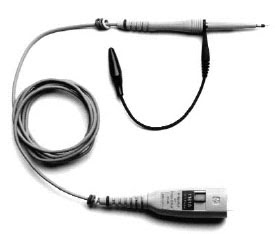Now we look at the probe tip of the scope probe. The figure below shows the zoom in view of the scope tip.Next, we dismantle the scope probe tip as shown in the figure below.Next we measure the probe tip resistance & capacitance. The equivalent probe tip schematic is shown below. From here we know that the probe tip resistance is 9Mohm and the probe tip capacitance is 10pF in parallel with the 9Mohm resistor.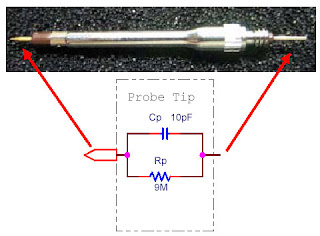Next, we move on to dismantle the probe connector. After removing the plastic casing, there is a small PCB with a metal shield.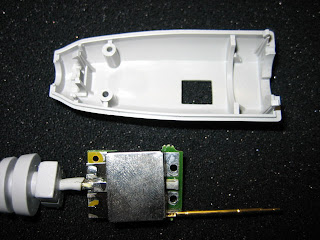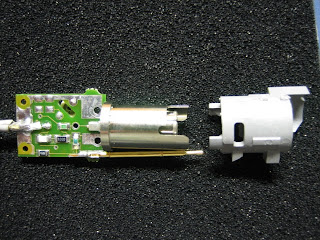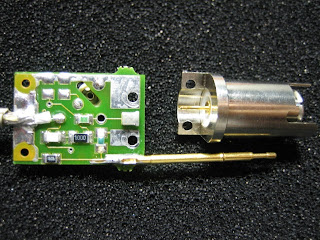Remove the metal shield and we could see the passive components now. There are a couple of SMT resistors and capacitors mounted on the PCB.Then we look at the opposite side of the PCB. There are two variable resistors and one variable capacitor on this side of PCB. The two variable resistors are used for high frequency compensation and the variable capacitor is use for low frequency compensation. We will show how these variable components works later.From here we could derive the probe compensation schematic inside the probe connector. Click the image below to view in enlarge format.From the schematic above, we have shown the probe tip, probe connector and oscilloscope circuit diagram. The typical oscilloscope input resistance is 1M ohm with a 12pF capacitance in parallel. We must include these resistor and capacitor into the calculation in order to obtain the correct compensation network calculation. R2 & R3 are the variable resistors (range of 0 to 500 Ohm) and C2 is the variable capacitor (Range of 50pF to 100pF).

We could simplify the schematic above into the circuit as shown below. Our objective is to calculate the relationship between Vin and Vscope across frequency from 100Hz to 100MHz.Z1 is the impedance of Cp and Rp in parallel. [ Z1= Rp // (1/sCp) ]

Z3 is the impedance of (C1 & R1 in series) and parallel with R2. [ Z3= (R1+ (1/sC1)) // R2 ]

Z4 is the impedance of C2 and R3 in series. [ Z4 = R3 + (1/sC2) ]

Z6 is the impedance of Rs and Cs in parallel. [ Z6 = Rs // (1 /sCs) ]

The table below shows the calculation of Vout versus Vin of 1V. As we can see, from 1Hz to 100kHz, the attenuation is 10 times. (Vout =1V /10=0.1V ). From 1M to 100M, the attenuation become lesser due to Z1 reduce steeply from 115k Ohm to less than 1k Ohm. This is the result of impedance Xcp has reduced as the frequency increase Xcp = 1/sCp ( s = 2*pi*freq).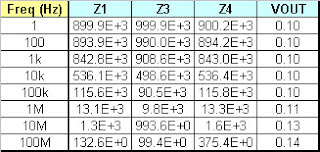We can say that with the passive components value as shown above, the scope probe has an accurate 10:1 attenuation from 1 Hz to 100kHz. From 1 MHz onwards, we need to fine tune the variable resistor R3 in order to obtain the 10:1 attenuation. Let change the R3 from 147 ohm to 50 ohm and check out the VOUT value now. It is clear that VOUT is almost consistenly 0.1 across all the frequency from 1Hz to high freq 100MHz.Conclusion:
1) Scope probe has compensation network to fine tune the VOUT value close to the attenuation value 10:1.
2) During the calculation of scope probe VOUT, we need to include the input resistor and capacitance of oscilloscope as well.